octal number systems in table 1-3. You can see that one octal digit is the equivalent value of three binary digits. The following examples of the conversion of octal 2258 to binary and back again further illustrate this comparison: ">Custom SearchOCTAL NUMBER SYSTEM The octal, or base 8, number system is a common system used with computers. Because of its relationship with the binary system, it is useful in programming some types of computers. Look closely at the comparison of binary and octal number systems in table 1-3. You can see that one octal digit is the equivalent value of three binary digits. The following examples of the conversion of octal 2258 to binary and back again further illustrate this comparison: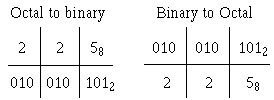Table 1-3. - Binary and Octal Comparison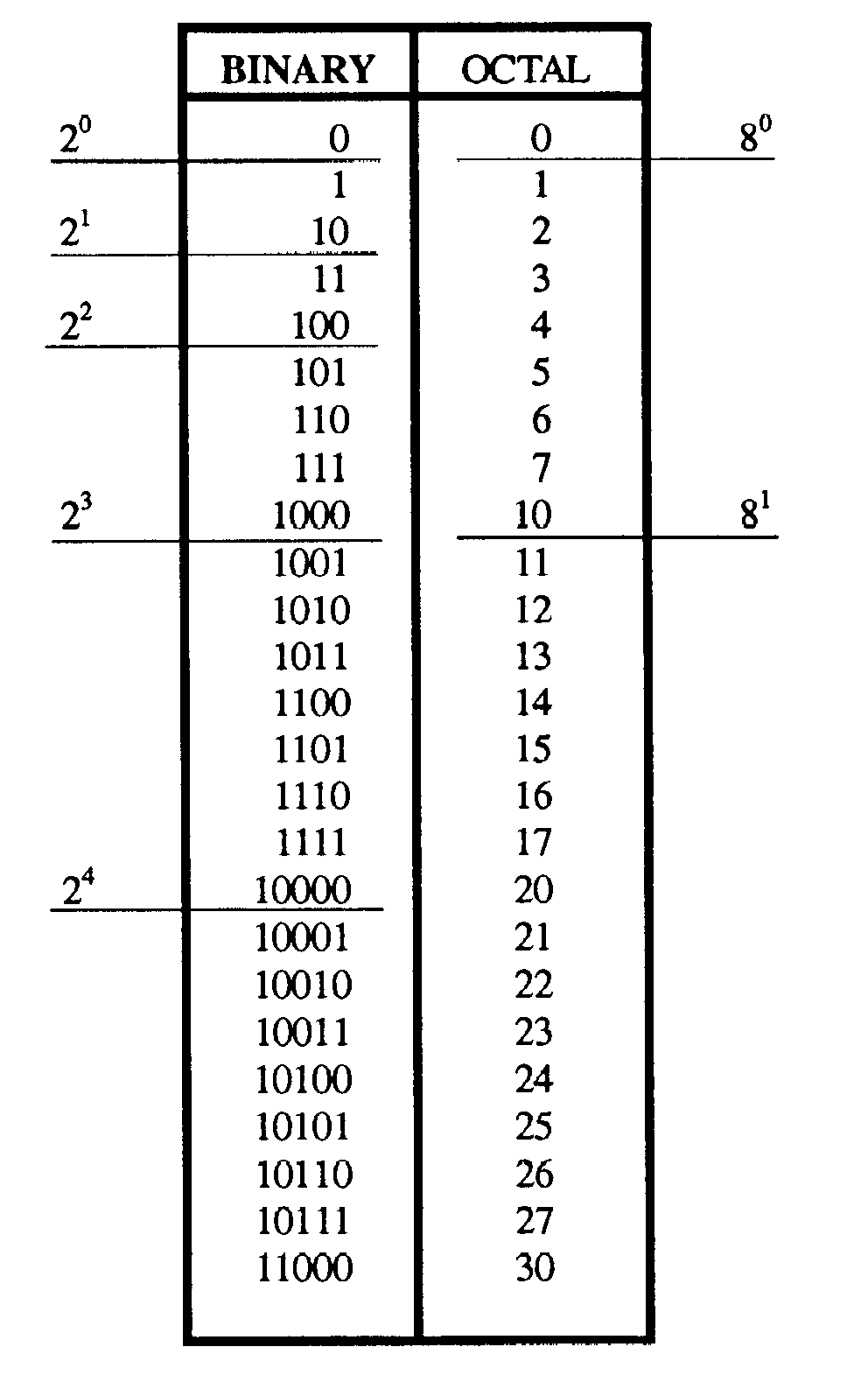Unit and Number The terms that you learned in the decimal and binary sections are also used with the octal system. The unit remains a single object, and the number is still a symbol used to represent one or more units. Base (Radix) As with the other systems, the radix, or base, is the number of symbols used in the system. The octal system uses eight symbols - 0 through 7. The base, or radix, is indicated by the subscript 8. Positional Notation The octal number system is a positional notation number system. Just as the decimal system uses powers of 10 and the binary system uses powers of 2, the octal system uses power of 8 to determine the value of a number's position. The following bar graph shows the positions and the power of the base: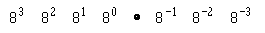Remember, that the power, or exponent, indicates the number of times the base is multiplied by itself. The value of this multiplication is expressed in base 10 as shown below: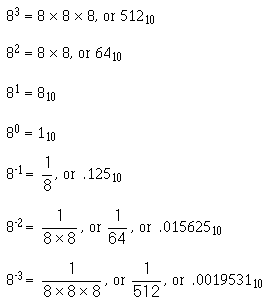All numbers to the left of the radix point are whole numbers, and those to the right are fractional numbers. MSD and LSD When determining the most and least significant digits in an octal number, use the same rules that you used with the other number systems. The digit farthest to the left of the radix point is the MSD, and the one farthest right of the radix point is the LSD. Example: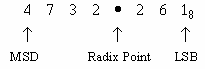If the number is a whole number, the MSD is the nonzero digit farthest to the left of the radix point and the LSD is the digit immediately to the left of the radix point. Conversely, if the number is a fraction only, the nonzero digit closest to the radix point is the MSD and the LSD is the nonzero digit farthest to the right of the radix point. Addition of Octal Numbers The addition of octal numbers is not difficult provided you remember that anytime the sum of two digits exceeds 7, a carry is produced. Compare the two examples shown below: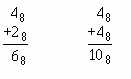The octal addition table in table 1-4 will be of benefit to you until you are accustomed to adding octal numbers. To use the table, simply follow the directions used in this example: Add: 68 and 58 Table 1-4. - Octal Addition Table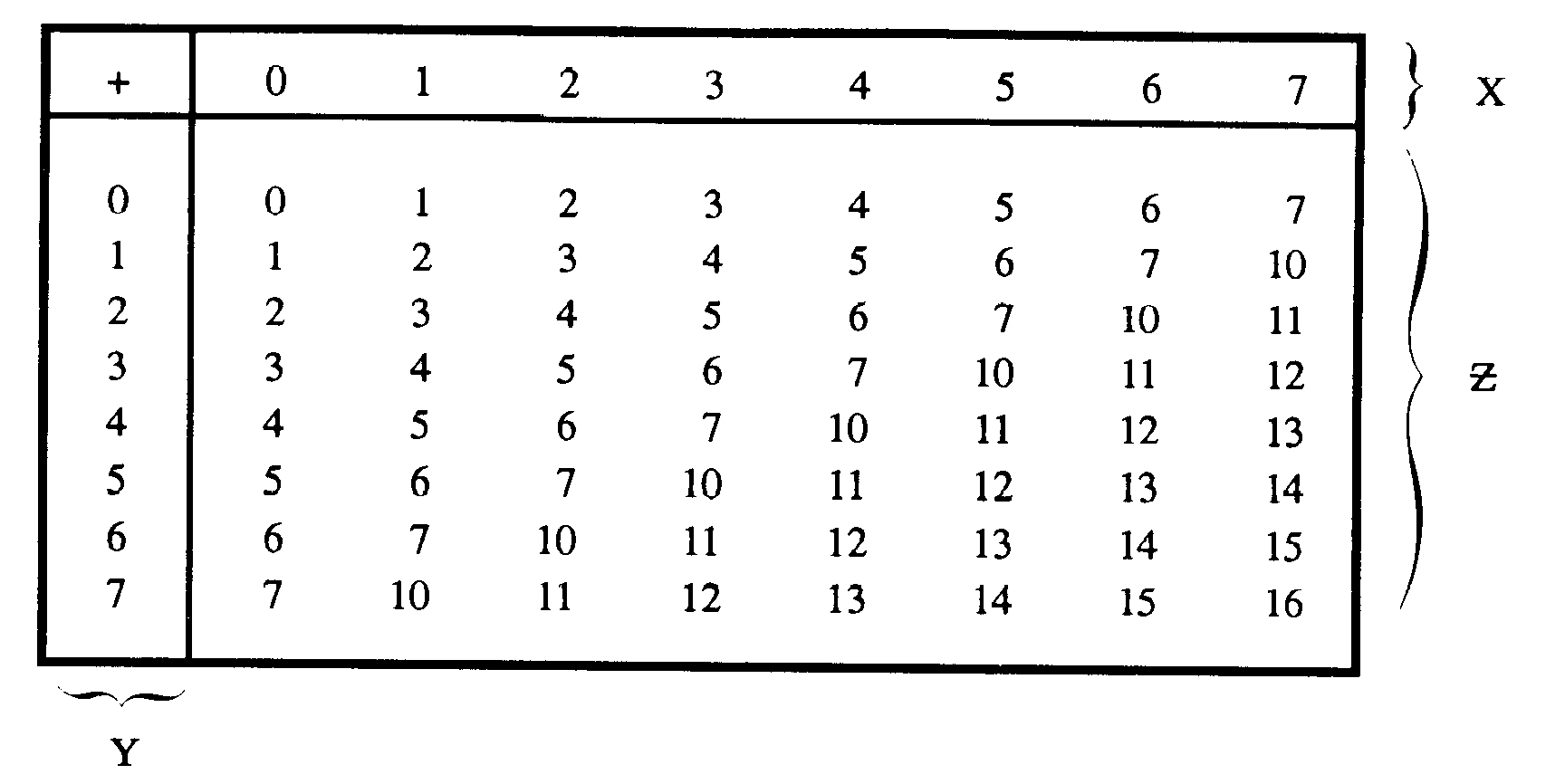Locate the 6 in the X column of the figure. Next locate the 5 in the Y column. The point in area Z where these two columns intersect is the sum. Therefore,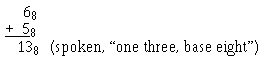If you use the concepts of addition you have already learned, you are ready to add octal numbers. Work through the solutions to the following problems: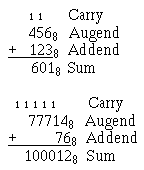As was mentioned earlier in this section, each time the sum of a column of numbers exceeds 7, a carry is produced. More than one carry may be produced if there are three or more numbers to be added, as in this example: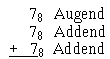The sum of the augend and the first addend is 68 with a carry. The sum of 68 and the second addend is 58 with a carry. You should write down the 58 and add the two carries and bring them down to the sum, as shown below: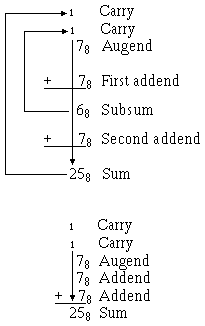Now let's try some practice problems: Q.24 Add: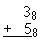Q.25 Add: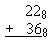Q.26 Add: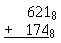Q.27 Add: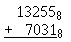Q.28 Add: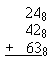Q.29 Add: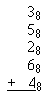Integrated Publishing, Inc. - A (SDVOSB) Service Disabled Veteran Owned Small Business